# DFT and FFT

### Getting started with DFT and FFT

The idea behind DFT and FFT is to get the frequency spectrum of a signal.
Frequency spectrum means that you can see which frequencies are inside a signal.
Remember that every signal can be described as a superposition of sine and cosine signals.

DFT/FFT is based on Correlation.
The DFT/FFT is a correlation between the given signal and a sin/cosine with a given frequency.

So if we have a look at the formula for the DFT we see: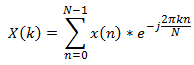that we correlate the given signal x with a set of sinus/cosine signals.

N is representing the number of samples.
The frequency band from 0 to fs(sampling frequency) is divided by N.
N will determine the frequency resolution we can achieve because you can see that we divide the exponent of e by N. The higher N is, the lower is the stepsize and therefore the higher the resolution as frequencies between the discrete N might be unrecognized.

If we want to achieve a specific frequency resolution we need to figure out how many samples we need at least: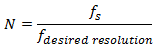At radix-2 the result is rounded to the next power of 2.

In return we can now calculate the Data collection time required: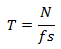We use the “twiddle factor” to introduce constant factors that can be precalculated.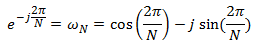in the DFT formula: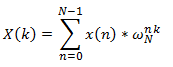Lets have a look at the twiddle factor a bit more precisely.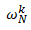Where N is the Number of “frequency steps” and k is the actual position within the unit circle.

Unit circle of N=8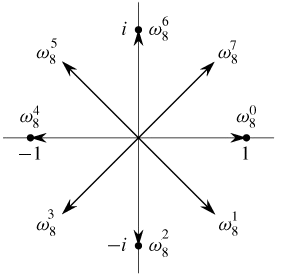With this knowledge we now can also see that
we can describe the same point in different ways: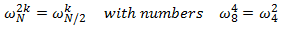we can use the symmetry of the cycle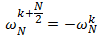And its periodicty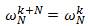The main problem with DFT is that we do need a lot of MAC operations: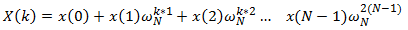When using the Fast Fourier Transform we want to make the DFT fast. By knowing about the periodicity and symmetry we now can reduce the amount of needed complex multiplications.
Also, instead of calculating each Twiddle factor over and over again we can precalculate all needed Factors.
In a nutshell FFT is the technology to avoid redundant calculations.

Derivation in Time

A radix-2 decimation-in-time (DIT) FFT is the simplest and most common form of the Cooley–Tukey algorithm […] Radix-2 DIT divides a DFT of size N into two interleaved DFTs (hence the name “radix-2”) of size N/2 with each recursive stage.[…]Radix-2 DIT first computes the DFTs of the even-indexed inputs and of the odd-indexed inputs and then combines those two results to produce the DFT of the whole sequence. This idea can then be performed recursively to reduce the overall runtime to O(N log N).

Wikipedia

So first we need to split our signal in even and odd parts: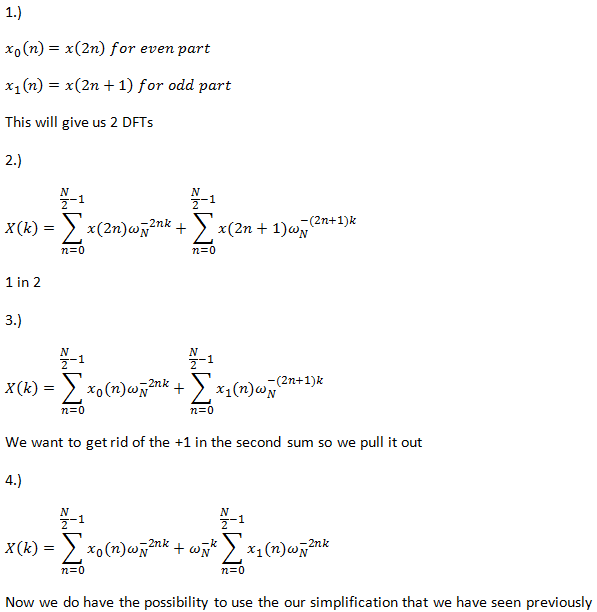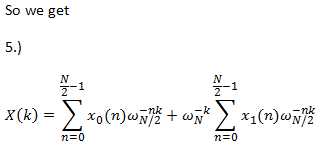Lets describe the 2 DFTs independent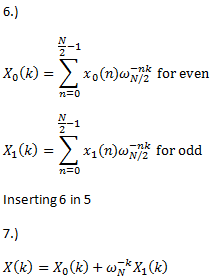The N/2 DFT of a signal is also a N/2 sequence. Moreover the DFT is periodic.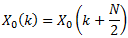Assuming an 8-point DFT we can now use this property to reduce the number of different coefficients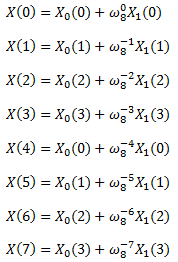And now comes the trick:
by usingwe get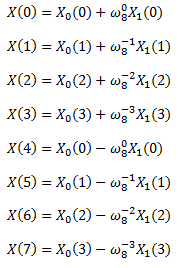As you can see a lot of computations are not necessary anymore because previous results can be reused. That is the core of radix-2.

#### Windowing

The problem here is that the DFT is just able to see a subset of the total signal. So if for example the set of samples is not meeting exactly the period of the signal.
This then can lead to so called spectral leakage as we simple “cut off” the signal and therefore produce very sharp edges.

The idea of windowing is to amplify the input signal in such a way that values close to the end and beginning of the value set are attenuated.
You can find some of the window functions here

This site uses cookies. By continuing to browse the site you are agreeing to our use of cookies.